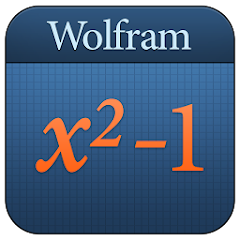# Algebra Course Assistant

10K+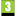PEGI 3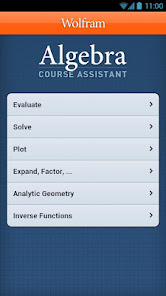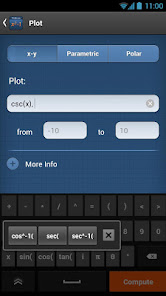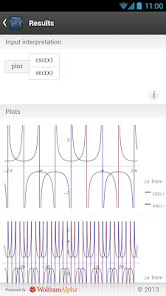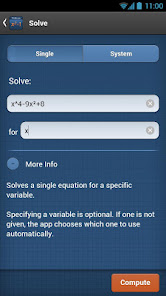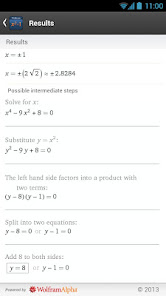Taking algebra? Then you need the Wolfram Algebra Course Assistant. This definitive app for algebra--from the world leader in math software--will help you quickly solve your homework problems, ace your tests, and learn algebra concepts so you're prepared for your next courses. Forget canned examples! The Wolfram Algebra Course Assistant solves your specific algebra problems on the fly, often showing you how to work through the problem step by step.

This app covers the following topics applicable to Algebra I, Algebra II, and College Algebra:
- Evaluate any numeric expression or substitute a value for a variable.
- Simplify fractions, square roots, or any other expression.
- Solve a simple equation or a system of equations for specific variables.
- Plot basic, parametric, or polar plots of the function(s) of your choice.
- Expand any polynomial.
- Factor numeric expressions, polynomials, and symbolic expressions.
- Divide any two expressions.
- Find the partial fraction decomposition of rational expressions.

The Wolfram Algebra Course Assistant is powered by the Wolfram|Alpha computational knowledge engine and is created by Wolfram Research, makers of Mathematica—the world's leading software system for mathematical research and education.

The Wolfram Algebra Course Assistant draws on the computational power of Wolfram|Alpha's supercomputers over a 2G, 3G, 4G, or Wi-Fi connection.
Updated on
Sep 11, 2018

## Data safety

Developers can show information here about how their app collects and uses your data. Learn more about data safetyNo information available

- Bug fixes